##### Question

In: Statistics and Probability

# In 1990, the mean height of women 20 years of age or older was 63.7 inches...

In 1990, the mean height of women 20 years of age or older was 63.7 inches based on data obtained from the CDC. Suppose that a random sample of 45 women who are 20 years of age or older in 2015 results in a mean height of 63.9 inches with a standard deviation of 0.5 inch. Does your sample provide sufficient evidence that women today are taller than in 1990? Perform the appropriate test at the 0.05 level of significance.

(a) Give the appropriate hypotheses for this test and define any parameters used.

(b) List and verify conditions for the appropriate hypothesis test.

(c) Give the appropriate test statistic. Show formula, work, and value.

(d) Find the rejection region. Draw an appropriate picture, show work.

(e) What is your decision regarding the null hypothesis?

## Solutions

##### Expert Solution

To Test :-

H0 :-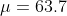H1 :-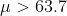Samples taken should be from normal population

Test Statistic :-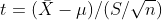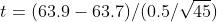t = 2.6833

Test Criteria :-
Reject null hypothesis if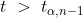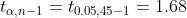Rejection region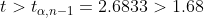Result :- Reject null hypothesis

Decision based on P value
P - value = P ( t > 2.6833 ) = 0.0051
Reject null hypothesis if P value <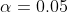level of significance
P - value = 0.0051 < 0.05 ,hence we reject null hypothesis
Conclusion :- Reject null hypothesis

There is sufficient evidence to support the claim that today's women re taller than 1990's at 5% level of significance.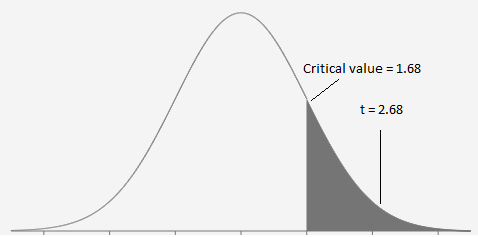## Related Solutions

##### 50 years ago, the mean height for women in their 20’s was 62.6 inches. Assume that...
50 years ago, the mean height for women in their 20’s was 62.6 inches. Assume that the heights of today’s women in their 20’s are approximately normally distributed with a standard deviation of 2.88 inches. If the mean height today is the same as that of a halfcentury ago, what percentage of all samples of size 25 today’s women have mean heights of at least 64.24 inches? please show work.
##### The mean height of women in a country​ (ages 20−​29) is 64.2 inches. A random sample...
The mean height of women in a country​ (ages 20−​29) is 64.2 inches. A random sample of 50 women in this age group is selected. What is the probability that the mean height for the sample is greater than 65​ inches? Assume σ equals=2.58. Round to four decimal places.
##### The height of women ages​ 20-29 is normally​ distributed, with a mean of 64.4 inches. Assume...
The height of women ages​ 20-29 is normally​ distributed, with a mean of 64.4 inches. Assume sigma = 2.6 inches. Are you more likely to randomly select 1 woman with a height less than 65.8 inches or are you more likely to select a sample of 22 women with a mean height less than 65.8 ​inches? Explain. What is the probability of randomly selecting 1 woman with a height less than 65.8 ​inches? What is the probability of selecting a...
##### The mean height of women in a country? (ages 20??29) is 63.6 inches. A random sample...
The mean height of women in a country? (ages 20??29) is 63.6 inches. A random sample of 50 women in this age group is selected. What is the probability that the mean height for the sample is greater than 64 ?inches? Assume ?=2.74 The probability that the mean height for the sample is greater than 64 inches is ___ ?
##### The height of women ages​ 20-29 is normally​ distributed, with a mean of 64.2 inches. Assume...
The height of women ages​ 20-29 is normally​ distributed, with a mean of 64.2 inches. Assume σ=2.7 inches. Are you more likely to randomly select 1 woman with a height less than 66.3 inches or are you more likely to select a sample of 14 women with a mean height less than 66.3​inches? Explain.
##### The height of women ages​ 20-29 are normally​ distributed, with a mean of 64.3 inches. Assume...
The height of women ages​ 20-29 are normally​ distributed, with a mean of 64.3 inches. Assume sigmaequals2.5 inches. Are you more likely to randomly select 1 woman with a height less than 66.2 inches or are you more likely to select a sample of 18 women with a mean height less than 66.2 ​inches? Explain. What is the probability of randomly selecting 1 woman with a height of less than 66.2 ​inches? _______​(Round to four decimal places as​ needed.) What...
##### The height of women ages​ 20-29 is normally​ distributed, with a mean of 64.2 inches. Assume...
The height of women ages​ 20-29 is normally​ distributed, with a mean of 64.2 inches. Assume sigmaequals2.9 inches. Are you more likely to randomly select 1 woman with a height less than 65.3 inches or are you more likely to select a sample of 29 women with a mean height less than 65.3 ​inches? Explain. LOADING... Click the icon to view page 1 of the standard normal table. LOADING... Click the icon to view page 2 of the standard normal...
##### The height of women ages​ 20-29 is normally​ distributed, with a mean of 64.7 inches. Assume...
The height of women ages​ 20-29 is normally​ distributed, with a mean of 64.7 inches. Assume sigmaσequals=2.7 inches. Are you more likely to randomly select 1 woman with a height less than 67.267.2 inches or are you more likely to select a sample of 10 women with a mean height less than 67.2 ​inches? Explain. LOADING... Click the icon to view page 1 of the standard normal table. LOADING... Click the icon to view page 2 of the standard normal...
##### The mean height of women in a country​ (ages 20-​29) is 64.1 inches. A random sample...
The mean height of women in a country​ (ages 20-​29) is 64.1 inches. A random sample of 70 women in this age group is selected. What is the probability that the mean height for the sample is greater than 65 ​inches? Assume σ=2.58. The probability that the mean height for the sample is greater than 65 inches is ______ . (Round to four decimal places as​ needed.)
##### 2. The mean height of American women in their twenties is about 64.3 inches, and the...
2. The mean height of American women in their twenties is about 64.3 inches, and the standard deviation is about 2.7 inches. The mean height of men the same age is about 69.9 inches, with standard deviation about 3.1 inches. Suppose that the correlation between the heights of husbands and wives is about r = 0.5. What are the slope and intercept of the regression line of the husband’s height on the wife’s height in young couples? Interpret the slope...